# Implementing DFA for no runs of length less than 4 for input (a,b)

• Last Updated : 16 Jun, 2022

DFA or Deterministic Finite Automata is a finite state machine, in which on every input alphabet a transition is done from one state to another state according to set of defined rules as per the need of string acceptance.
In this particular problem, runs of length is the factor to think about.The input alphabets are {a, b}. No runs of length less than 4 means any input alphabets repeats itself minimum 4 times.

Example:

Input: n = “aaabab”
Output: string not accepted
Explanation: The starting letter a is not repeated at least 4 times. Hence DFA failed.

Input: n = “aaaabbbbaaaabbbbbaaaaa”
Output: string accepted
Explanation: Every unique occurrence of a and b are repeated at least 4 times. Hence DFA passed.

Designing DFA step by step:
Step-1: First we have to think about what input alphabet is inputted. As this is about minimum 4 runs of length. Take input alphabet is ‘a’ on state “A” and mark this state as initial state. The following transition occur on input ‘a’:

• From state “A” to state “B”
• From state “B” to state “C”
• From state “C” to state “D”
• From state “D” to state “E”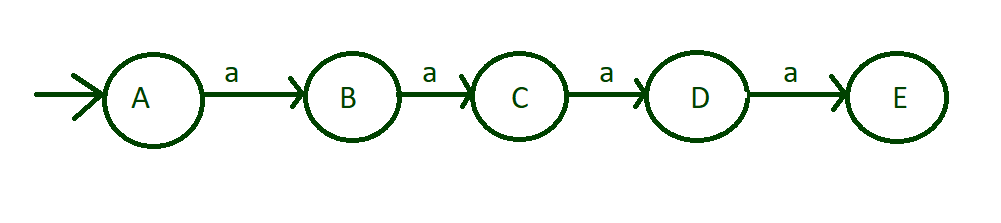input of run of length 4

Step-2: As in above step, minimum 4 a’s are inputted which is acceptable so make state “E” is final state. Also runs of length more than 4 is acceptable so put self loop of ‘a’ on final state “E”.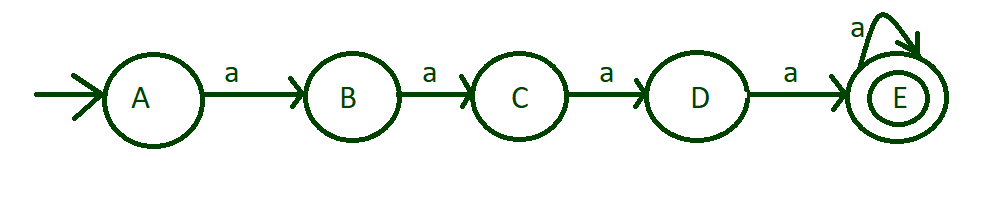marks state “E” to final state

Step-3: Till now machine design is done with input alphabet ‘a’.But input alphabet ‘b’ of state “A” is left to deal with. Now do the same process with input alphabet ‘b’ on state “A”.The following transition occur on input ‘b’:

• From state “A” to state “F”
• From state “F” to state “G”
• From state “G” to state “H”
• From state “H” to state “Q”

Mark state “Q” to final state. And also runs of length more than 4 is also acceptable so put self loop on final state “Q”.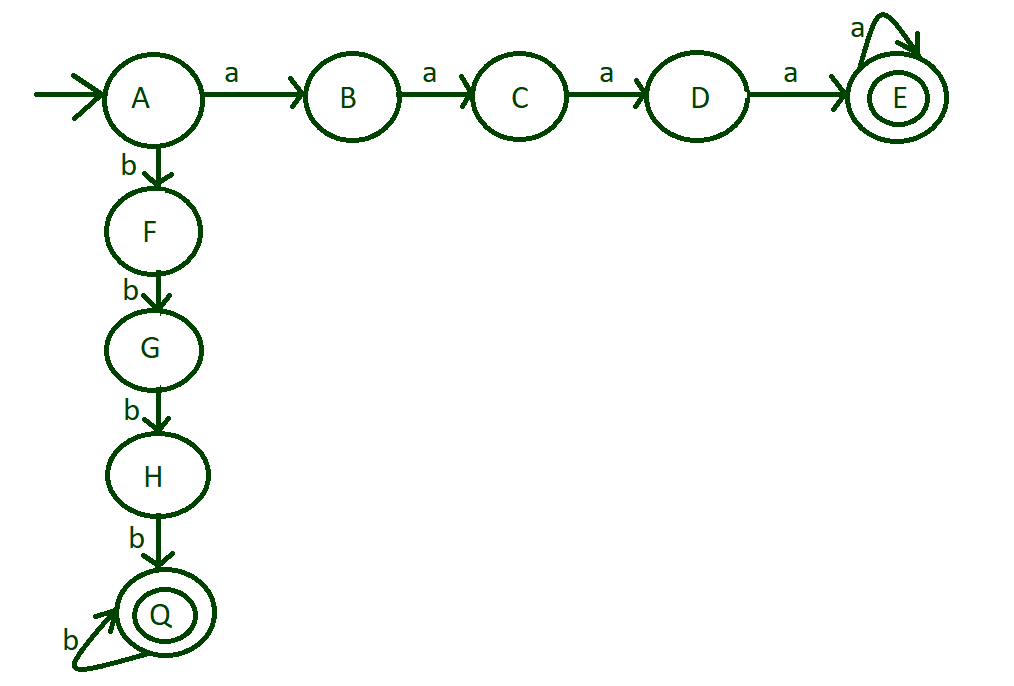input alphabet ‘b’ transitions

Step-4: Till now we have done with only single input a or b, i.e, aaaa, and bbbb, but now deal with both input such as aaaabbbb or aaaabbbbaaaaa etc.
For this transition of input ‘b’ from state “E” to state “F” and transition of input ‘a’ from state “Q” to state “B”.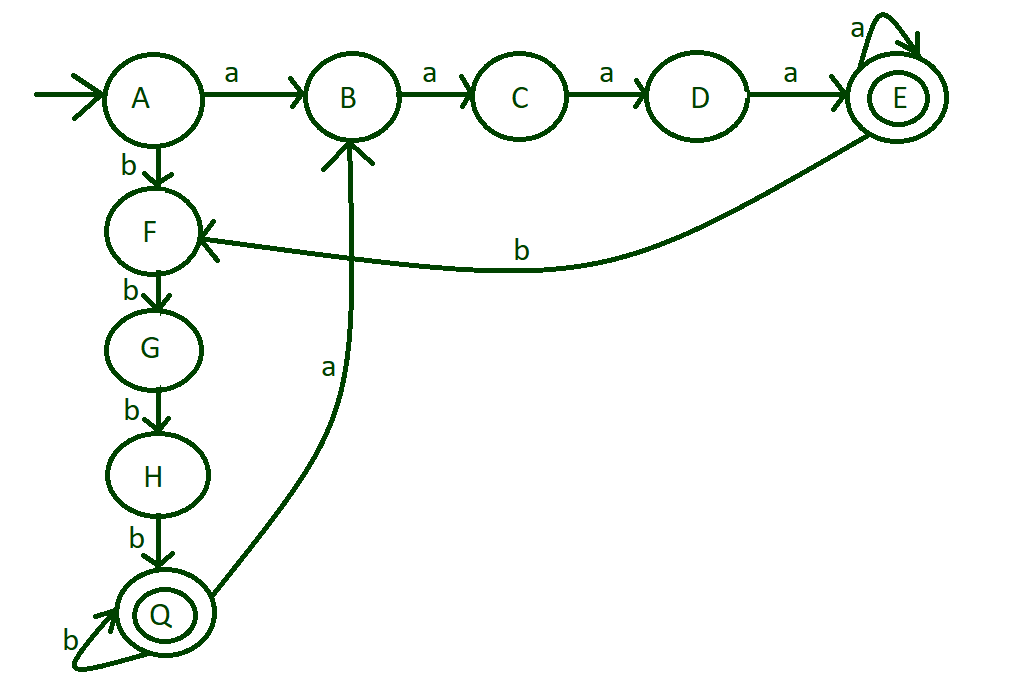Step-5: Now dealing with remaining input alphabets, till now designed machine have covered all possible acceptable cases and nor remaining input alphabets will go to dead state”DS”.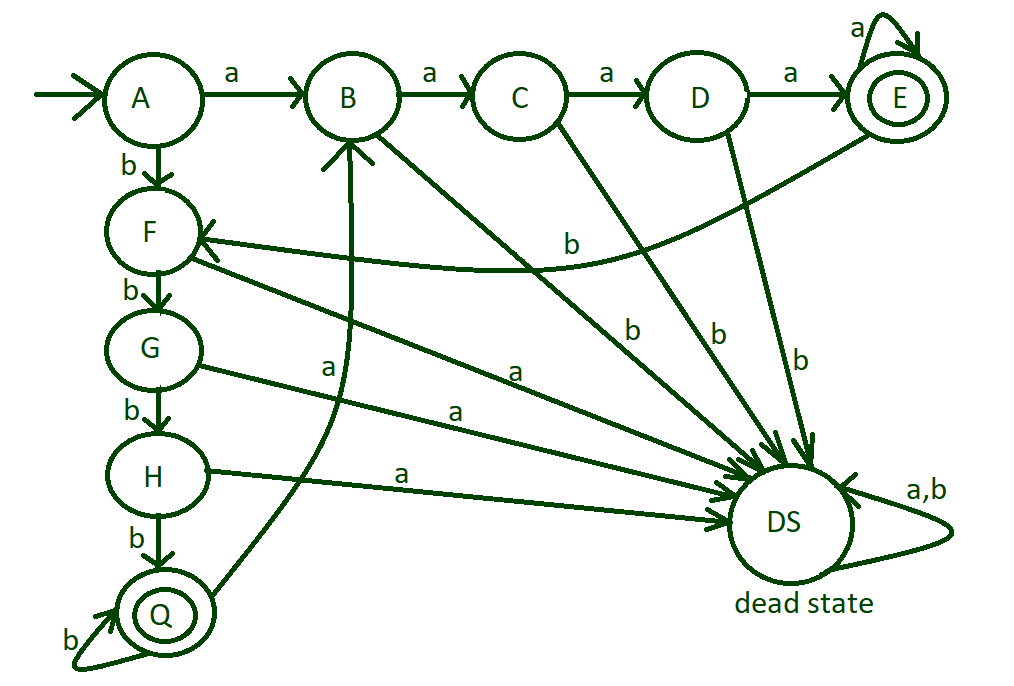final design

Transition Table of above DFA:
Here “A” is initial state and “E” and “Q” are final states. DS is termed as DEAD STATE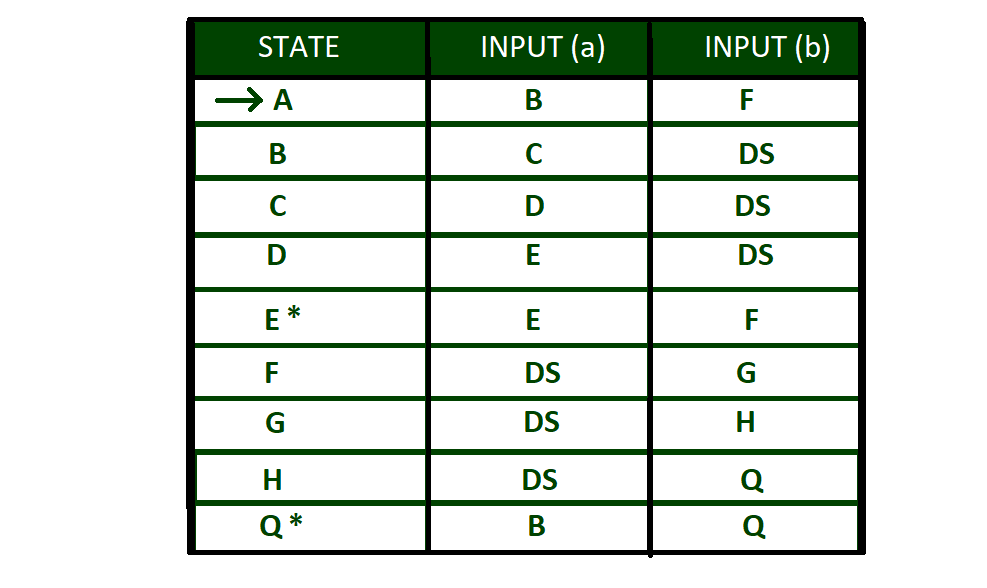Transition Table

Python implementation of above DFA:

## Python3

 `def` `checkStateA(n):` `    ``# if length of is 0` `    ``# print string not accepted` `    ``if``(``len``(n)``=``=` `0``):` `        ``print``(``"string not accepted"``)` `        `  `    ``else``:` `        ``# if 'a' is found ` `        ``# call stateB function` `        ``# else call stateF` `        ``# function` `        ``if``(n[``0``]``=``=``'a'``):` `            ``stateB(n[``1``:])` `        ``else``:` `            ``stateF(n[``1``:])` `    `    `def` `stateB(n):` `    ``# if length of is 0` `    ``# print string not accepted` `    ``if` `(``len``(n)``=``=` `0``):` `        ``print``(``"string not accepted"``)` `        `  `    ``else``:    ` `        ``# if 'a' is found ` `        ``# call stateC function` `        ``# print string not` `        ``# accepted` `        ``if``(n[``0``]``=``=``'a'``):` `            ``stateC(n[``1``:])` `        ``else``:` `            ``print``(``"string not accepted"``)` `    `  `    `  `def` `stateC(n):` `    ``# if length of is 0` `    ``# print string not accepted` `    ``if` `(``len``(n)``=``=` `0``):` `        ``print``(``"string not accepted"``)` `        `  `    ``else``:   ` `        ``# if 'a' is found ` `        ``# call stateD function` `        ``# print string not` `        ``# accepted` `        ``if``(n[``0``]``=``=``'a'``):` `            ``stateD(n[``1``:])` `        ``else``:` `            ``print``(``"string not accepted"``)` `    `  `    `  `def` `stateD(n):` `    ``# if length of is 0` `    ``# print string not accepted` `    ``if``(``len``(n)``=``=` `0``):` `        ``print``(``"string not accepted"``)` `        `  `    ``else``:    ` `        ``# if 'a' is found ` `        ``# call stateE function` `        ``# print string not` `        ``# accepted` `        ``if``(n[``0``]``=``=``'a'``):` `            ``stateE(n[``1``:])` `        ``else``:` `            ``print``(``"string not accepted"``)` `        `  `        `  `def` `stateE(n):` `    ``# if length of is 0` `    ``# print string accepted` `    ``if``(``len``(n)``=``=` `0``):` `        ``print``(``"string accepted"``)` `        `  `    ``# if 'a' is found ` `    ``# call stateE function` `    ``# if 'b' is found ` `    ``# call stateF function    ` `    ``elif``(n[``0``]``=``=``'a'``):` `        ``stateE(n[``1``:])` `    ``elif``(n[``0``]``=``=``'b'``):` `        ``stateF(n[``1``:])` `        `  `        `  `def` `stateF(n):` `    ``# if length of is 0` `    ``# print string not accepted` `    ``if``(``len``(n)``=``=` `0``):` `        ``print``(``"string not accepted"``)` `        `  `    ``else``: ` `        ``# if 'b' is found ` `        ``# call stateG function` `        ``# print string not` `        ``# accepted` `        ``if``(n[``0``]``=``=``'b'``):` `            ``stateG(n[``1``:])` `        ``else``:` `            ``print``(``"string not accepted"``)` `    `  `    `  `def` `stateG(n):` `    ``# if length of is 0` `    ``# print string not accepted` `    ``if``(``len``(n)``=``=` `0``):` `        ``print``(``"string not accepted"``)` `        `  `    ``else``:    ` `        ``# if 'b' is found ` `        ``# call stateHfunction` `        ``# print string not` `        ``# accepted` `        ``if``(n[``0``]``=``=``'b'``):` `            ``stateH(n[``1``:])` `        ``else``:` `            ``print``(``"string not accepted"``)` `            `  `            `  `def` `stateH(n):` `    ``# if length of is 0` `    ``# print string not accepted` `    ``if``(``len``(n)``=``=` `0``):` `        ``print``(``"string not accepted"``)` `        `  `    ``else``:  ` `        ``# if 'b' is found ` `        ``# call stateQ function` `        ``# print string not` `        ``# accepted` `        ``if``(n[``0``]``=``=``'b'``):` `            ``stateQ(n[``1``:])` `        ``else``:` `            ``print``(``"string not accepted"``)  ` `            `  `            `  `def` `stateQ(n):` `    ``# if length of is 0` `    ``# print string accepted` `    ``if``(``len``(n)``=``=` `0``):` `        ``print``(``"string accepted"``)` `        `  `    ``else``:   ` `        ``# if 'b' is found ` `        ``# call stateQ function` `        ``# else call stateB function` `        ``if``(n[``0``]``=``=``'b'``):` `            ``stateQ(n[``1``:])` `        ``elif``(n[``0``]``=``=``'a'``):` `            ``stateB(n[``1``:])            ` `    `  `        `  `        `    `# Driver code` `if` `__name__ ``=``=` `'__main__'``:`   `    ``# input string 1` `    ``n ``=``"aaaabbbbbaaaa"`   `    ``# function call to check the string` `    ``checkStateA(n)`   `    ``# input string 2` `    ``n ``=``"aaaabbb"`   `    ``# function call to check the string` `    ``checkStateA(n)`

Output:

```string accepted
string not accepteed```

My Personal Notes arrow_drop_up
Recommended Articles
Page :